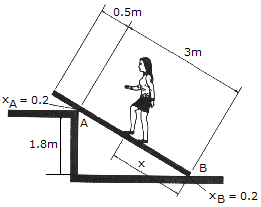# Engineering Mechanics - Friction - Discussion

### Discussion :: Friction - General Questions (Q.No.2)

2.A uniform beam has a mass of 18 kg and rests on two surfaces at points A and B. Determine the maximum distance x to which the girl can slowly walk up the beam before it begins to slip. The girl has a mass of 50 kg and walks up the beam with a constant velocity.

 [A]. x = 0.678 m [B]. x = 0.508 m [C]. x = 1.005 m [D]. x = 0.712 m

Explanation:

No answer description available for this question.

 Shubham said: (Mar 5, 2011) Please clearify the solution in a easy manner. Thanks.

 Raveesha said: (Jun 7, 2011) Please give the solution.

 Laxman said: (Feb 26, 2015) Please solve this problem step by step sir.

 Ani... said: (May 28, 2015) Pleas give the complete explanation briefly.

 Filip said: (Jul 22, 2015) 1st: draw the fbd. 2nd: Summation of Fx and Fy. 3rd: We now have 2 unknowns; Na and Nb. 4th: Use your calculator to get the unknowns. 5th: Sum of moment at B using the beam as the x-axis. Answer: 0.678 m.

 Shyam said: (Sep 9, 2015) I can't understand this problem please explain simple logically.

 Nikhil said: (Dec 30, 2016) I did not understand. Please explain it.

 Raj said: (Sep 18, 2019) Well said, thanks @Filip.

 Raj said: (Sep 18, 2019) Well said, thanks @Filip.

 Raj said: (Sep 18, 2019) Well said, thanks @Filip.

 Pranavjathrey said: (Aug 10, 2020) @All The solution is; Let θ be the internal angle at B sinθ = 1.8/3.0 sinθ = 0.6 θ = 36.87. At B, the normal force of the floor on the ramp Nb is vertical. At A, the normal force of the step on the ramp Na is perpendicular to the ramp or θ from vertical. Sum horizontal forces to zero. Assume right is the positive direction. Na(sinθ) - μ(Na)cosθ - μNb = 0. Na(sinθ - μcosθ) = μNb. Nb = Na(0.6 - 0.2(0.8)/0.2. Nb = 2.2Na. Sum vertical forces to zero. Assume up is positive. Nb + Na(cosθ) + μ(Na)sinθ - (M + m)g = 0. 2.2Na + Na(cosθ) + μ(Na)sinθ - (M + m)g = 0, Na (2.2 + cosθ + μsinθ) = (M + m)g, Na = (M + m)g / (2.2 + cosθ + μsinθ), Na = (50 + 18)9.81 / (2.2 + 0.8 + 0.2(0.6)), Na = 213.8. Sum moments about B to zero. Assume clockwise moment is positive'. Nb and the friction force at A have no moment arm so create no moment about B. Na[3.0] - Mg[xcosθ] - mg[(3.5 / 2)cosθ] = 0. x = (3Na - mg[1.75cosθ])/Mgcosθ. x = (3(213.8) - 18(9.81)1.75(0.8))/50(9.81)0.8. x = 1.005 m.

 Jayanta said: (Mar 13, 2021) @Pranavjathrey. Well done, thanks.